# Selina Solutions Concise Mathematics Class 6 Chapter 2 Estimation

Step by step Selina Solutions are given here for students of Class 6. These Selina Solutions help students to understand thoroughly the difficult questions while solving them. Subject experts have designed the solutions in order to help students in improving their performance in academics. Students who want to score high marks are advised to follow these solutions and practice them continuously during exam preparation.

Estimation means to make an idea about quantities, to judge approximate size, cost, population, etc. The problems of this chapter will improve students’ skills in solving in an effective way. Students can make use of the PDF of Selina Solutions Concise Mathematics Class 6 and download from the links available here.

## Selina Solutions Concise Mathematics Class 6 Chapter 2: Estimation Download PDF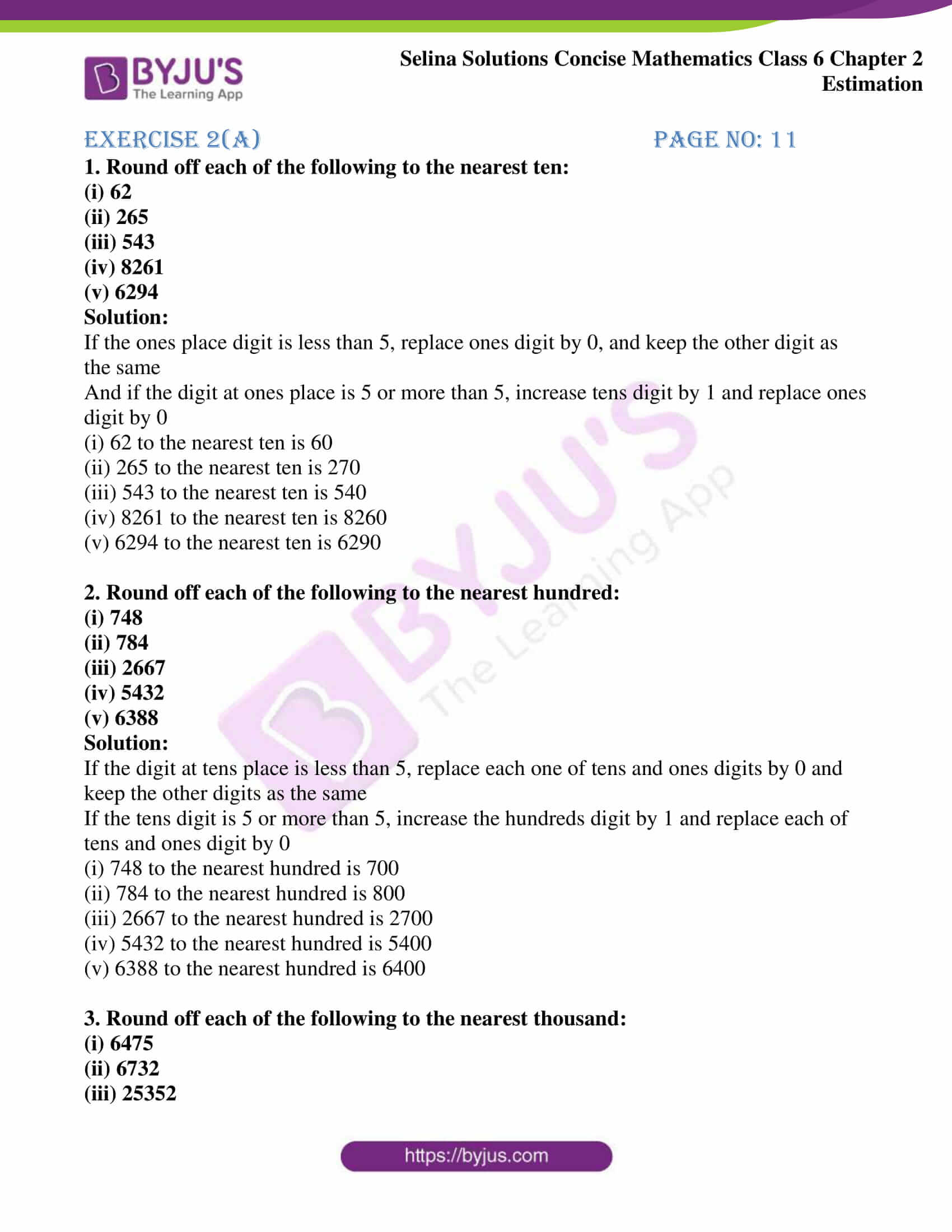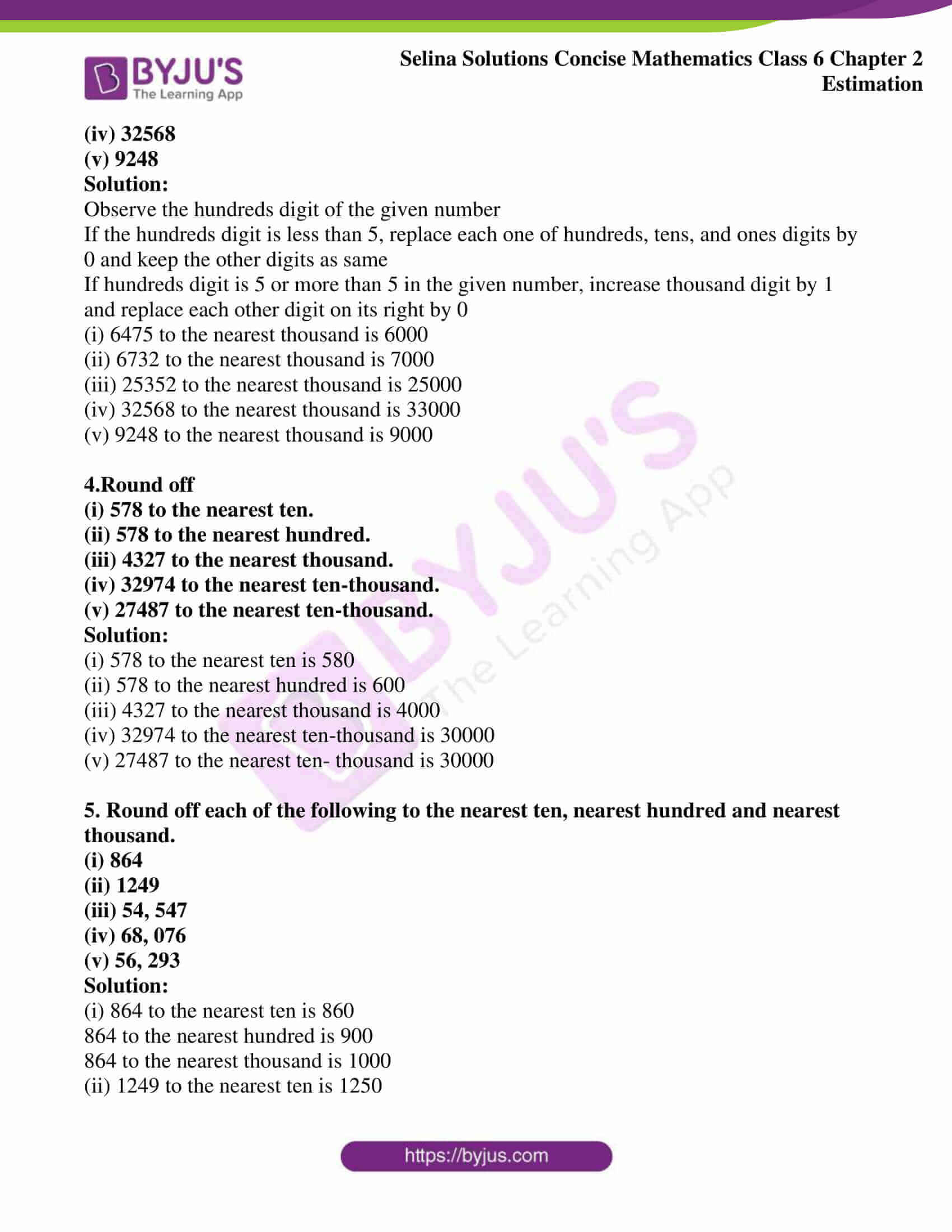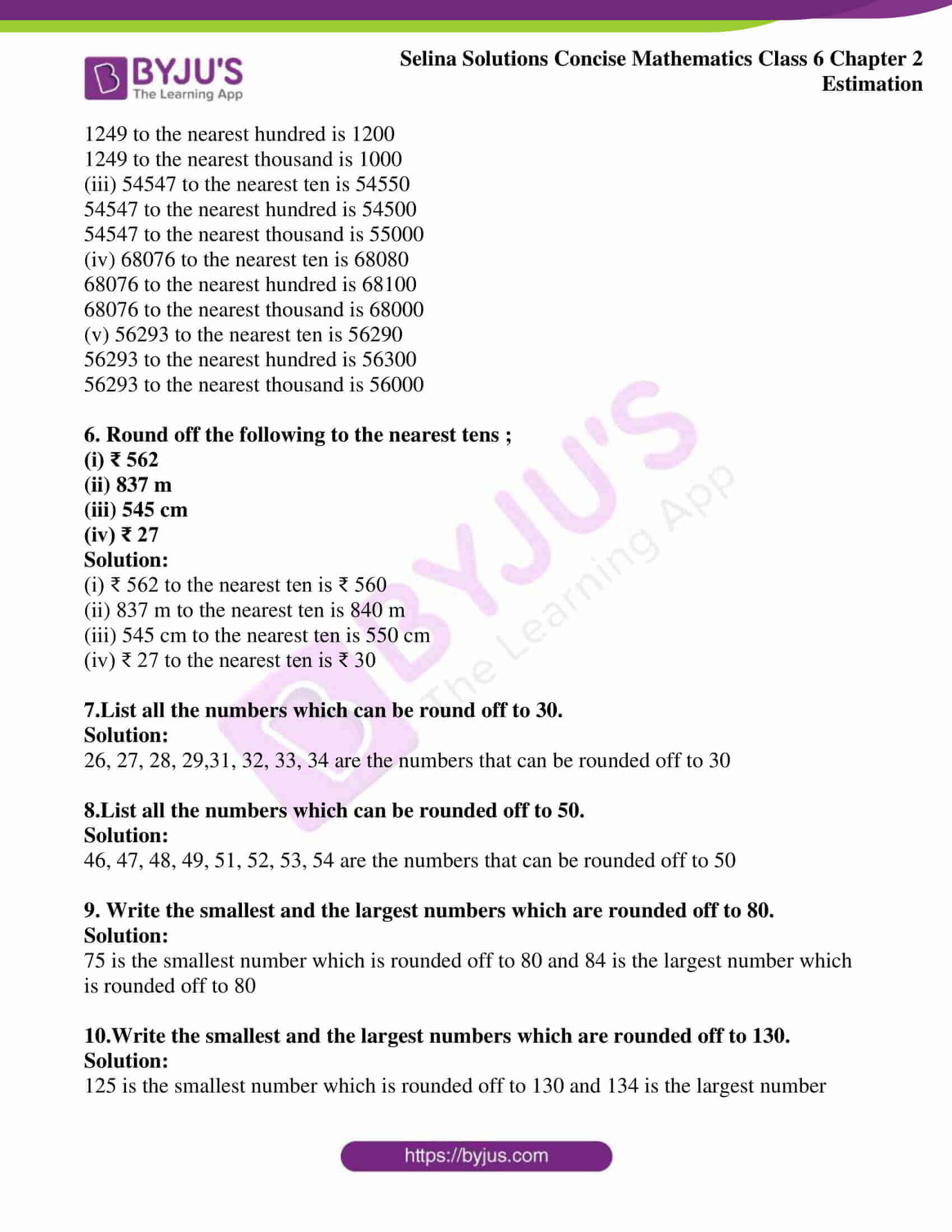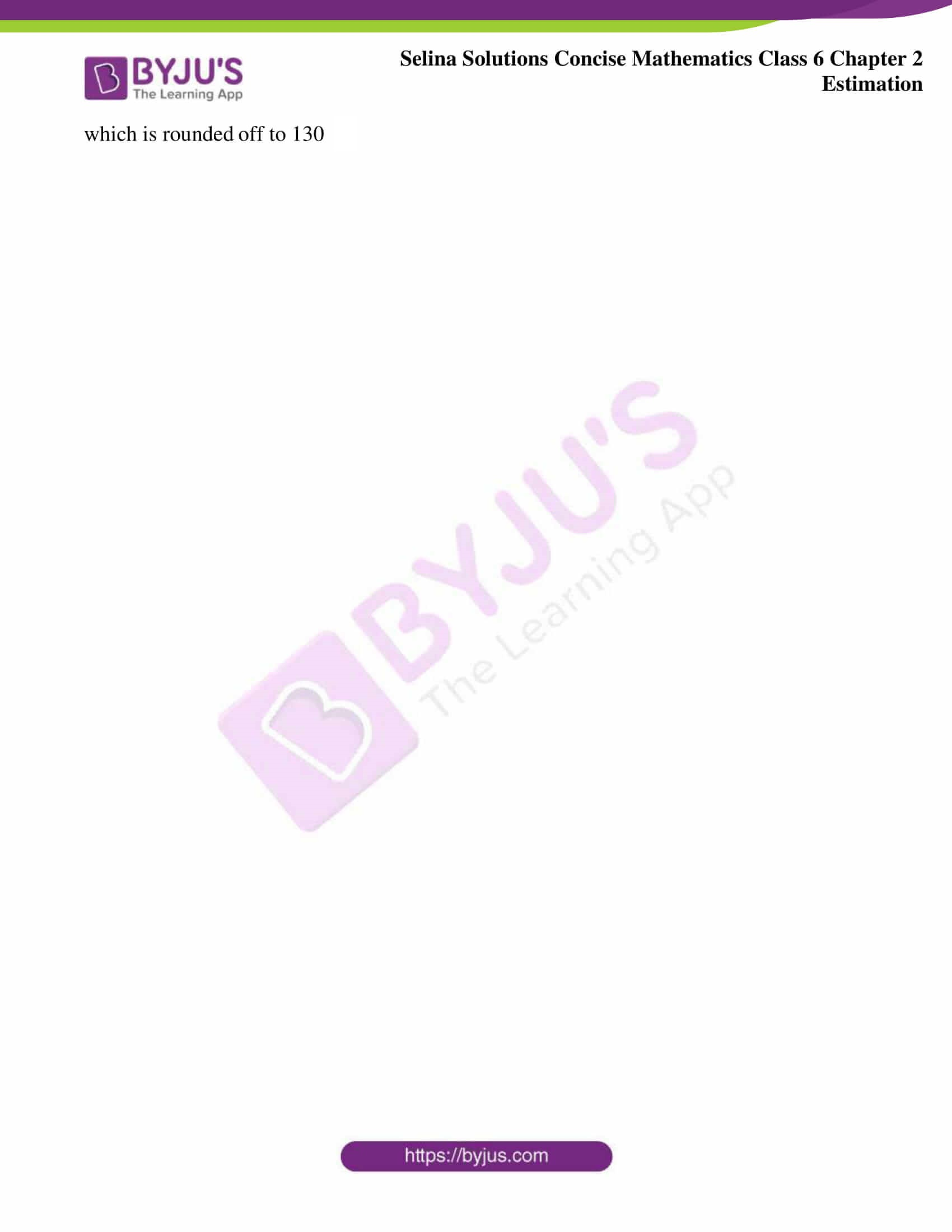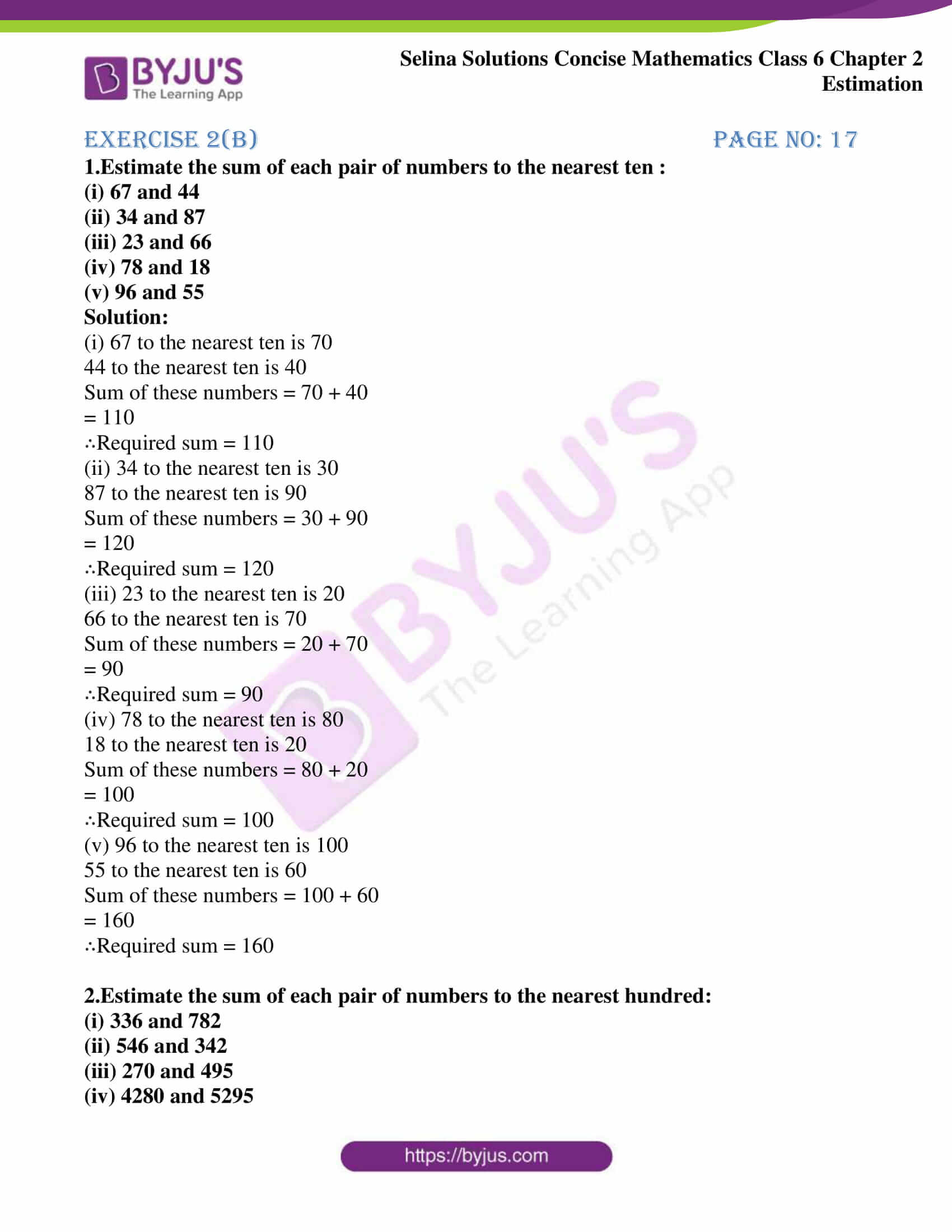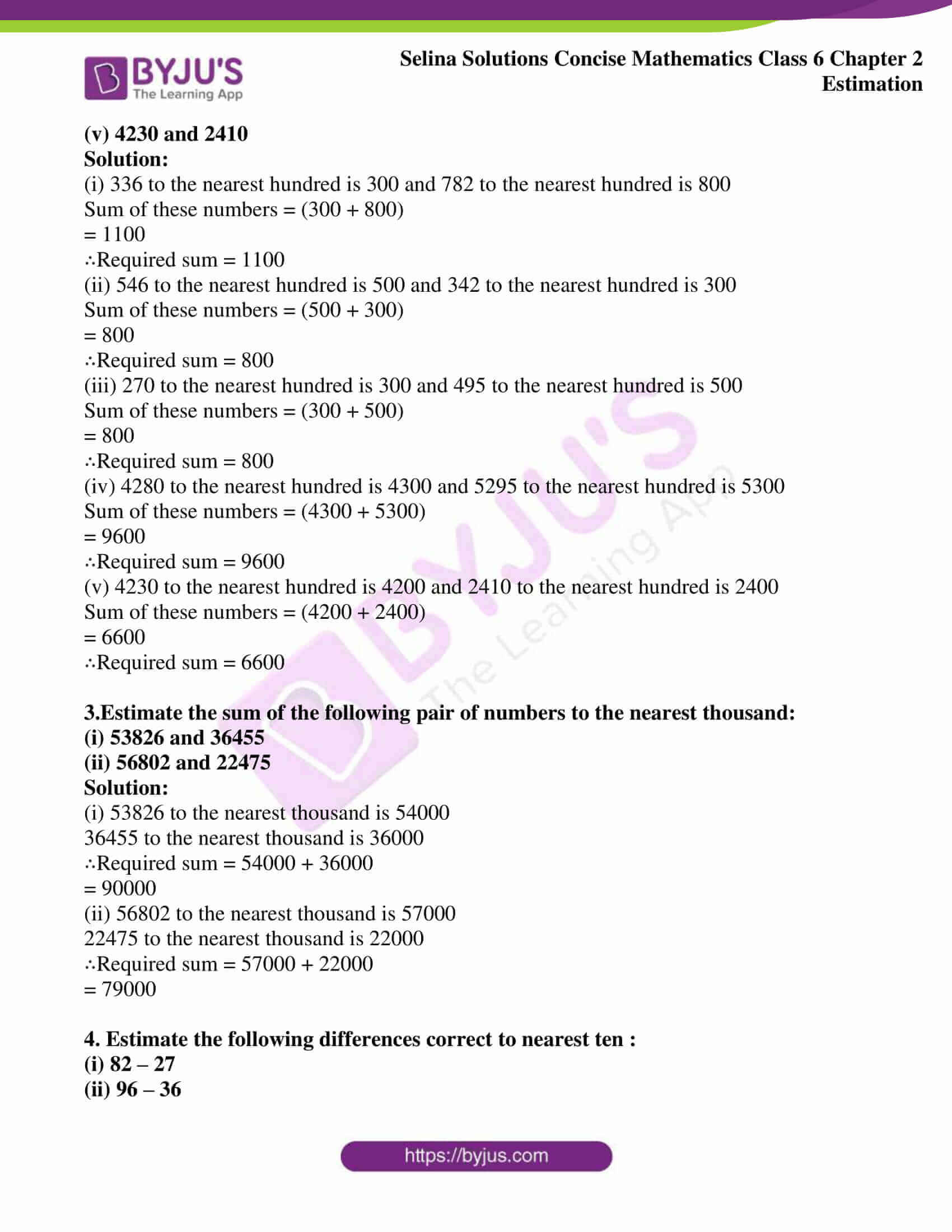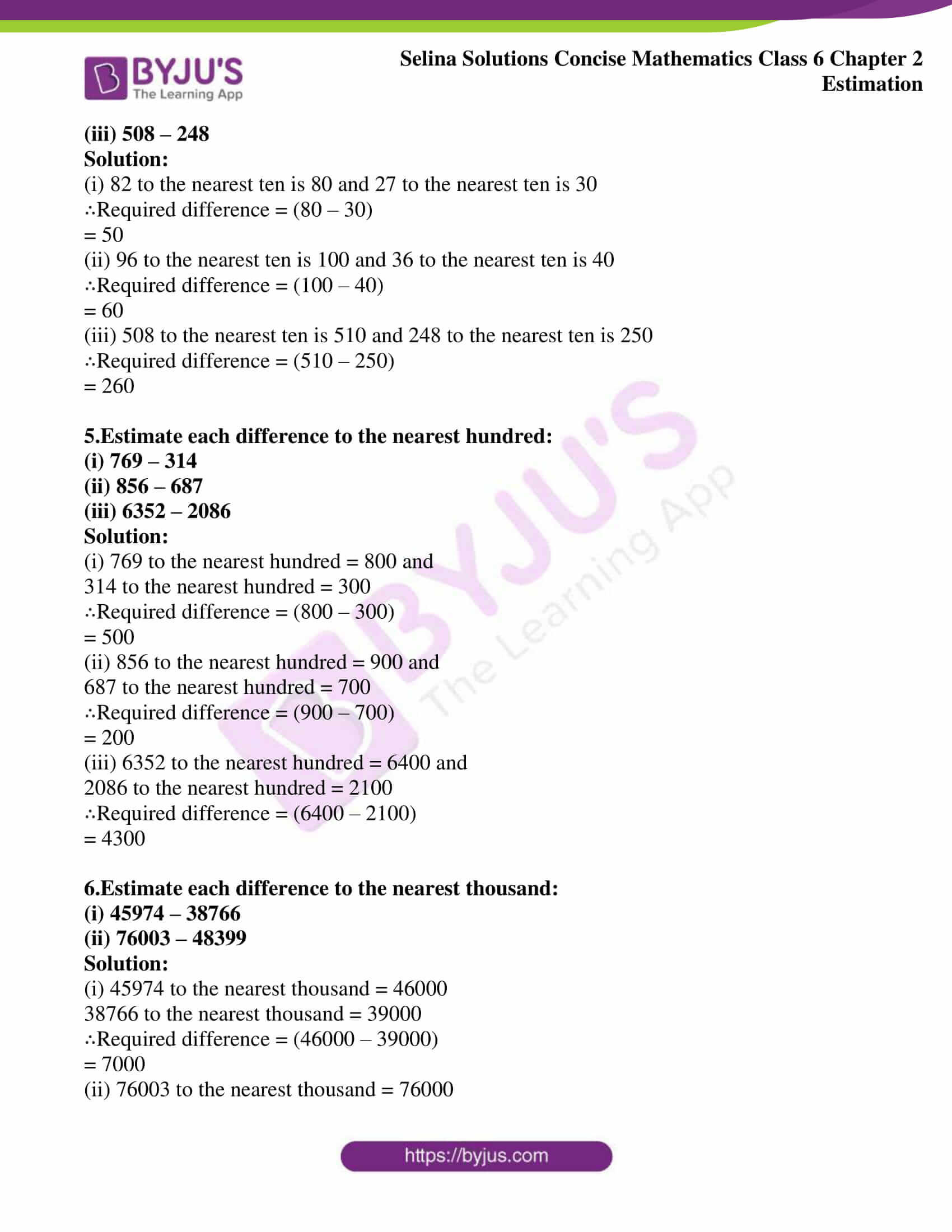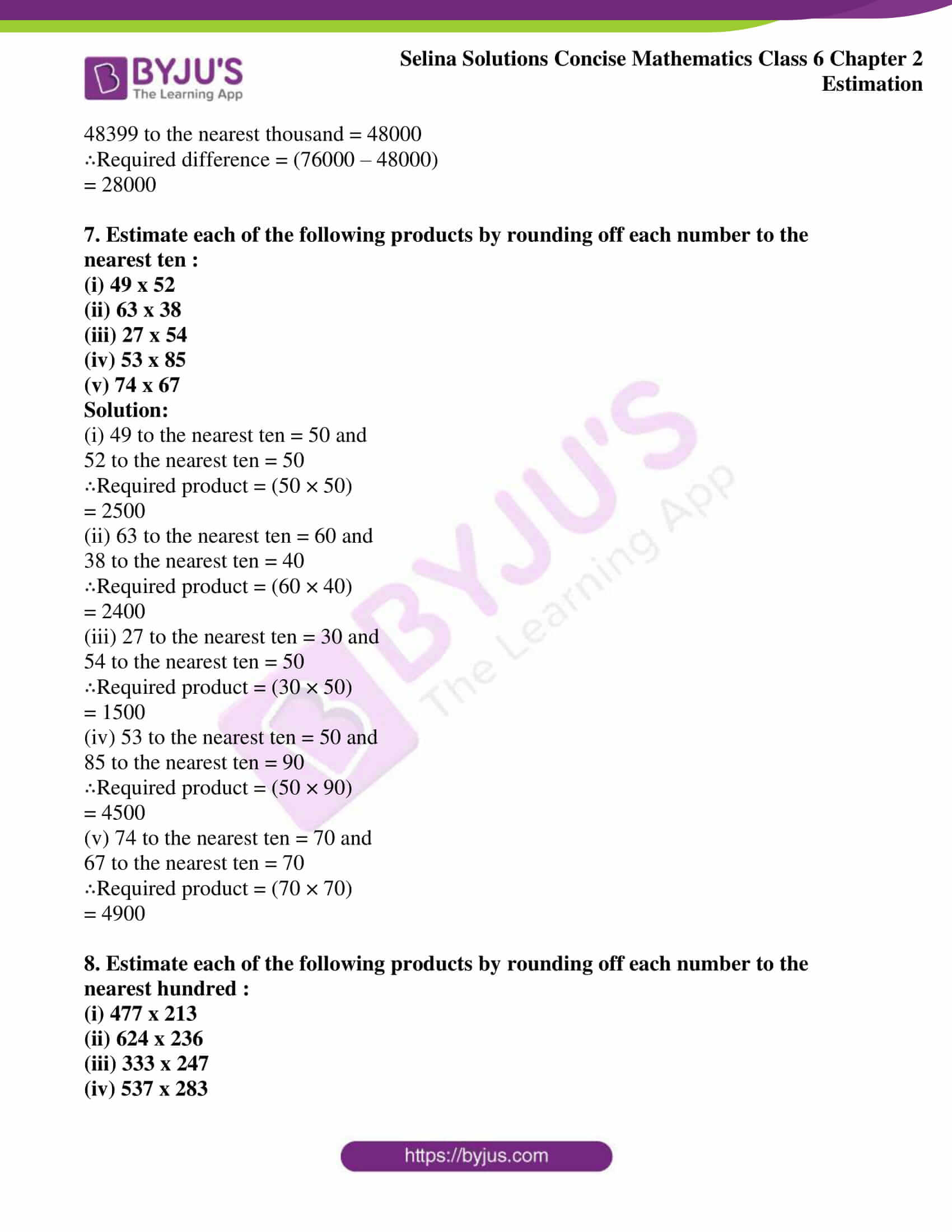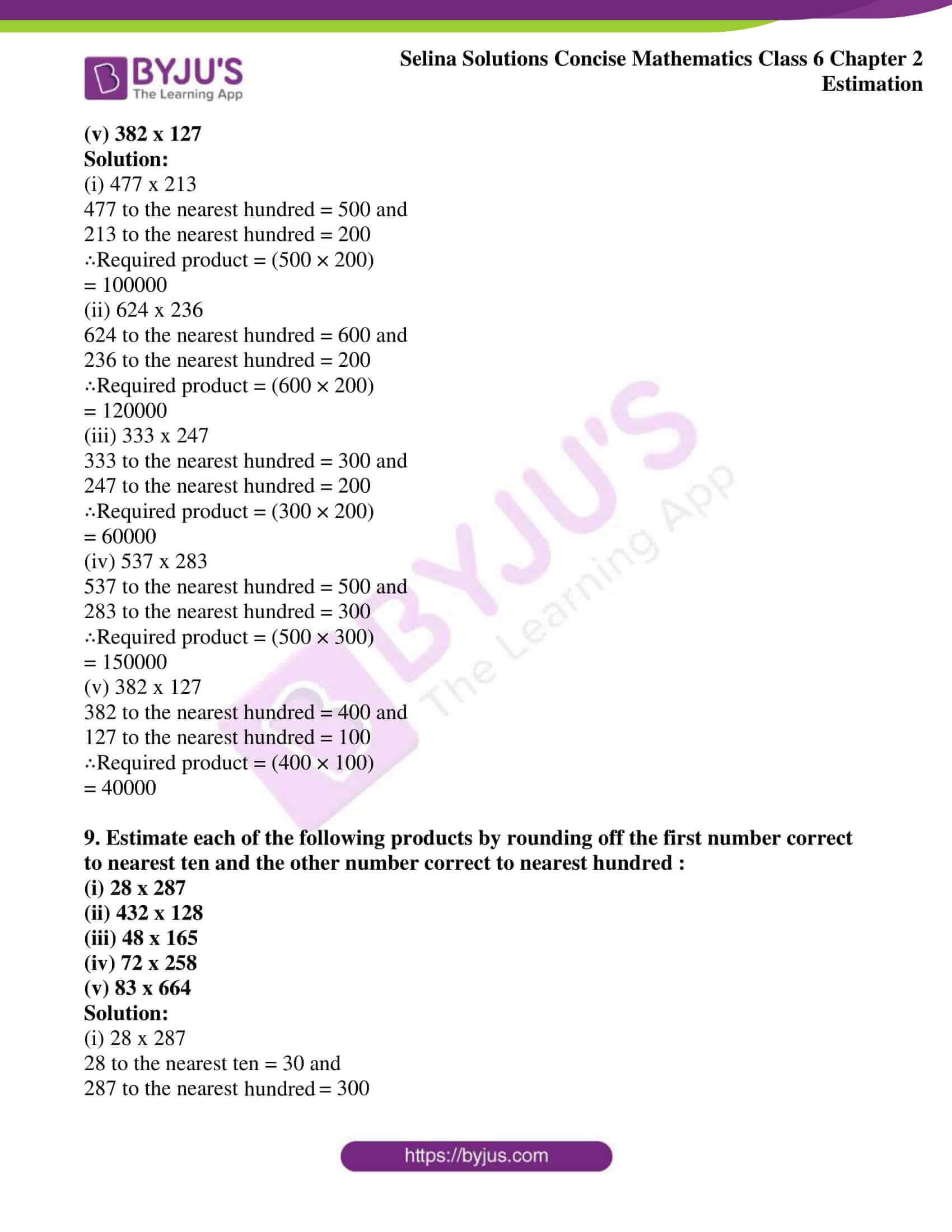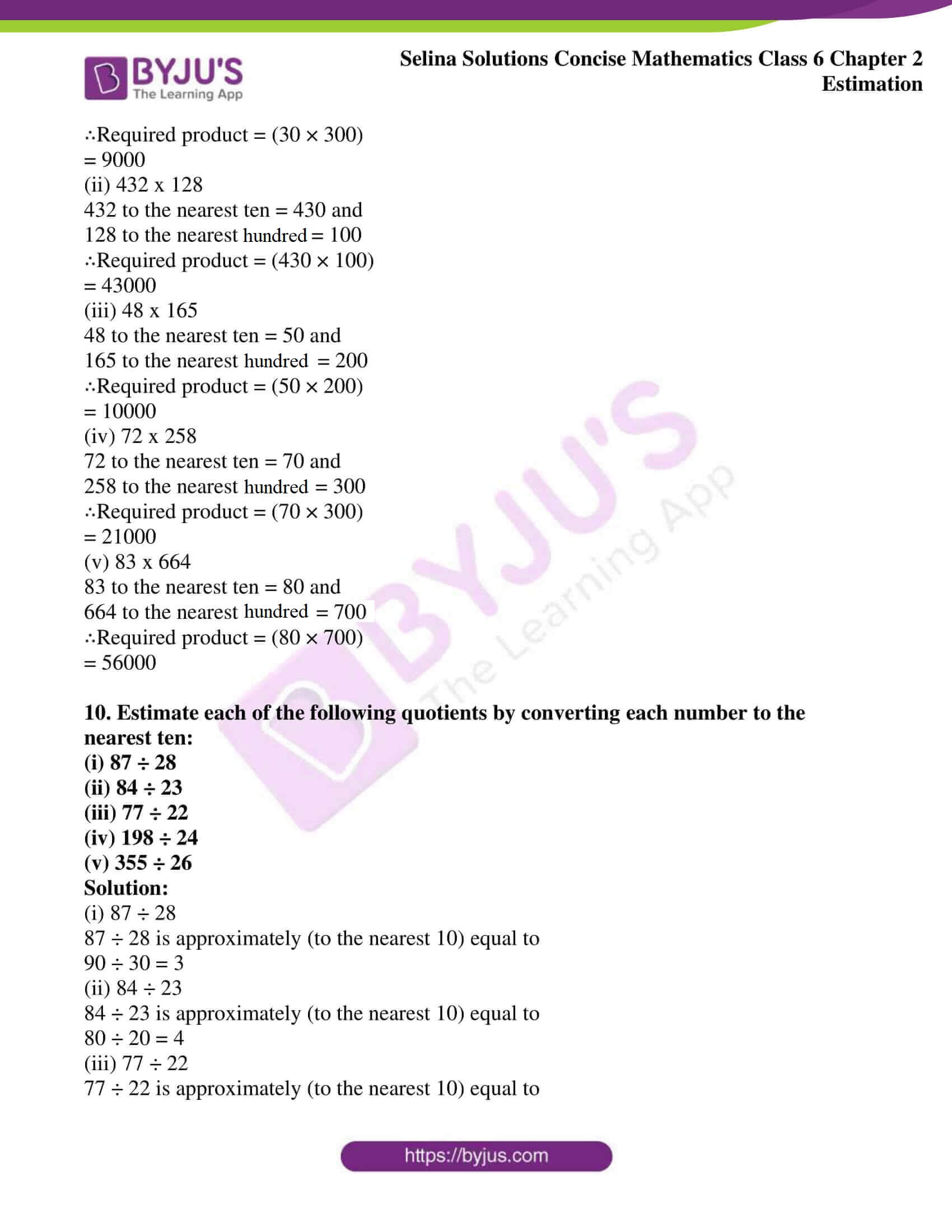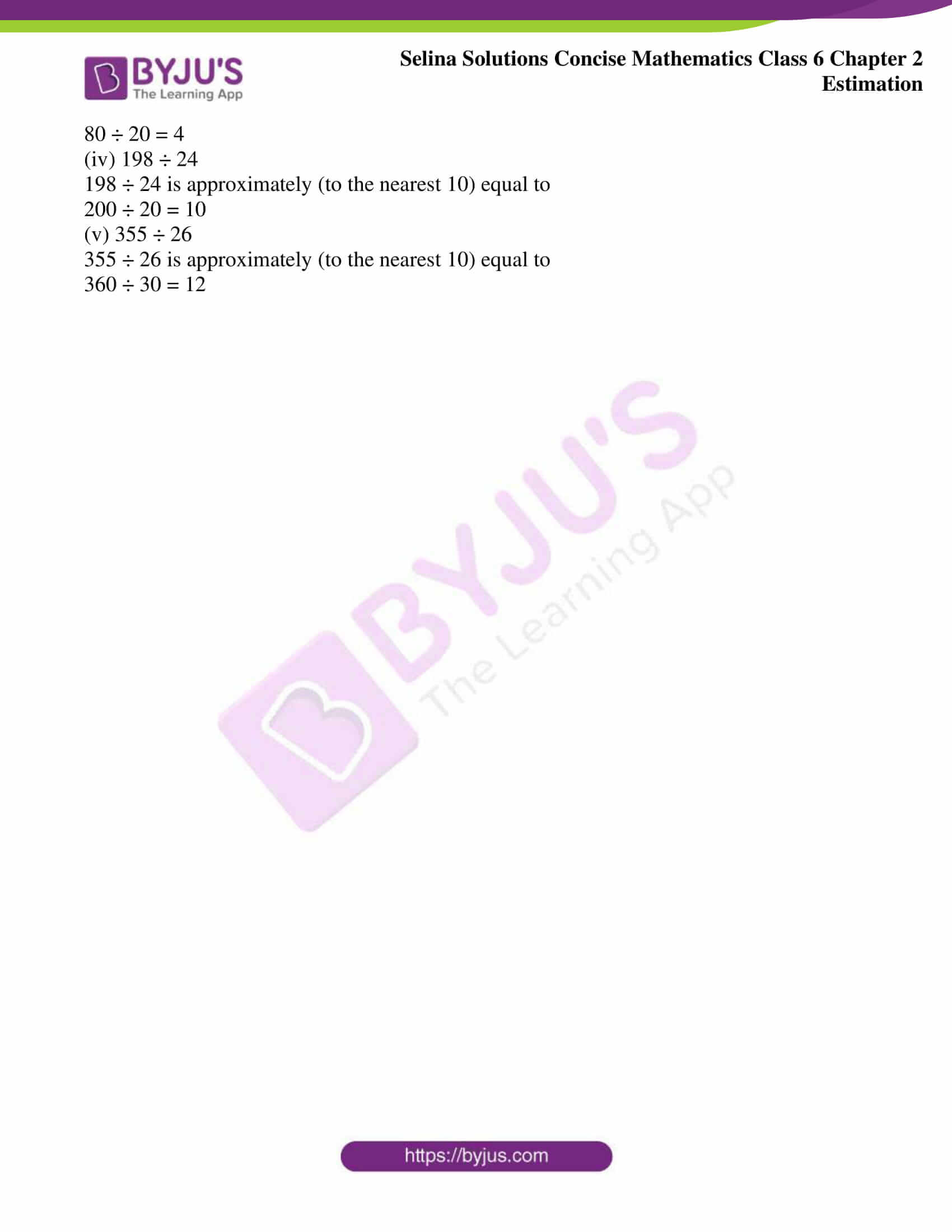## Exercises of Selina Solutions Concise Mathematics Class 6 Chapter 2: Estimation

Exercise 2(A) Solutions

Exercise 2(B) Solutions

### Access Selina Solutions Concise Mathematics Class 6 Chapter 2: Estimation

#### Exercise 2(A) page no: 11

1. Round off each of the following to the nearest ten:

(i) 62

(ii) 265

(iii) 543

(iv) 8261

(v) 6294

Solution:

If the ones place digit is less than 5, replace ones digit by 0, and keep the other digit as the same

And if the digit at ones place is 5 or more than 5, increase tens digit by 1 and replace ones digit by 0

(i) 62 to the nearest ten is 60

(ii) 265 to the nearest ten is 270

(iii) 543 to the nearest ten is 540

(iv) 8261 to the nearest ten is 8260

(v) 6294 to the nearest ten is 6290

2. Round off each of the following to the nearest hundred:

(i) 748

(ii) 784

(iii) 2667

(iv) 5432

(v) 6388

Solution:

If the digit at tens place is less than 5, replace each one of tens and ones digits by 0 and keep the other digits as the same

If the tens digit is 5 or more than 5, increase the hundreds digit by 1 and replace each of tens and ones digit by 0

(i) 748 to the nearest hundred is 700

(ii) 784 to the nearest hundred is 800

(iii) 2667 to the nearest hundred is 2700

(iv) 5432 to the nearest hundred is 5400

(v) 6388 to the nearest hundred is 6400

3. Round off each of the following to the nearest thousand:
(i) 6475
(ii) 6732
(iii) 25352
(iv) 32568
(v) 9248

Solution:

Observe the hundreds digit of the given number

If the hundreds digit is less than 5, replace each one of hundreds, tens, and ones digits by 0 and keep the other digits as same

If hundreds digit is 5 or more than 5 in the given number, increase thousand digit by 1 and replace each other digit on its right by 0

(i) 6475 to the nearest thousand is 6000

(ii) 6732 to the nearest thousand is 7000

(iii) 25352 to the nearest thousand is 25000

(iv) 32568 to the nearest thousand is 33000

(v) 9248 to the nearest thousand is 9000

4.Round off
(i) 578 to the nearest ten.
(ii) 578 to the nearest hundred.
(iii) 4327 to the nearest thousand.
(iv) 32974 to the nearest ten-thousand.
(v) 27487 to the nearest ten-thousand.

Solution:

(i) 578 to the nearest ten is 580

(ii) 578 to the nearest hundred is 600

(iii) 4327 to the nearest thousand is 4000

(iv) 32974 to the nearest ten-thousand is 30000

(v) 27487 to the nearest ten- thousand is 30000

5. Round off each of the following to the nearest ten, nearest hundred and nearest thousand.
(i) 864
(ii) 1249
(iii) 54, 547
(iv) 68, 076
(v) 56, 293

Solution:

(i) 864 to the nearest ten is 860

864 to the nearest hundred is 900

864 to the nearest thousand is 1000

(ii) 1249 to the nearest ten is 1250

1249 to the nearest hundred is 1200

1249 to the nearest thousand is 1000

(iii) 54547 to the nearest ten is 54550

54547 to the nearest hundred is 54500

54547 to the nearest thousand is 55000

(iv) 68076 to the nearest ten is 68080

68076 to the nearest hundred is 68100

68076 to the nearest thousand is 68000

(v) 56293 to the nearest ten is 56290

56293 to the nearest hundred is 56300

56293 to the nearest thousand is 56000

6. Round off the following to the nearest tens ;
(i) ₹ 562
(ii) 837 m
(iii) 545 cm
(iv) ₹ 27

Solution:

(i) ₹ 562 to the nearest ten is ₹ 560

(ii) 837 m to the nearest ten is 840 m

(iii) 545 cm to the nearest ten is 550 cm

(iv) ₹ 27 to the nearest ten is ₹ 30

7.List all the numbers which can be round off to 30.

Solution:

26, 27, 28, 29,31, 32, 33, 34 are the numbers that can be rounded off to 30

8.List all the numbers which can be rounded off to 50.
Solution:

46, 47, 48, 49, 51, 52, 53, 54 are the numbers that can be rounded off to 50

9. Write the smallest and the largest numbers which are rounded off to 80.

Solution:

75 is the smallest number which is rounded off to 80 and 84 is the largest number which is rounded off to 80

10.Write the smallest and the largest numbers which are rounded off to 130.

Solution:

125 is the smallest number which is rounded off to 130 and 134 is the largest number which is rounded off to 130

#### Exercise 2(b) page no: 17

1.Estimate the sum of each pair of numbers to the nearest ten :
(i) 67 and 44
(ii) 34 and 87
(iii) 23 and 66
(iv) 78 and 18
(v) 96 and 55

Solution:

(i) 67 to the nearest ten is 70

44 to the nearest ten is 40

Sum of these numbers = 70 + 40

= 110

∴Required sum = 110

(ii) 34 to the nearest ten is 30

87 to the nearest ten is 90

Sum of these numbers = 30 + 90

= 120

∴Required sum = 120

(iii) 23 to the nearest ten is 20

66 to the nearest ten is 70

Sum of these numbers = 20 + 70

= 90

∴Required sum = 90

(iv) 78 to the nearest ten is 80

18 to the nearest ten is 20

Sum of these numbers = 80 + 20

= 100

∴Required sum = 100

(v) 96 to the nearest ten is 100

55 to the nearest ten is 60

Sum of these numbers = 100 + 60

= 160

∴Required sum = 160

2.Estimate the sum of each pair of numbers to the nearest hundred:
(i) 336 and 782
(ii) 546 and 342
(iii) 270 and 495
(iv) 4280 and 5295

(v) 4230 and 2410

Solution:

(i) 336 to the nearest hundred is 300 and 782 to the nearest hundred is 800

Sum of these numbers = (300 + 800)

= 1100

∴Required sum = 1100

(ii) 546 to the nearest hundred is 500 and 342 to the nearest hundred is 300

Sum of these numbers = (500 + 300)

= 800

∴Required sum = 800

(iii) 270 to the nearest hundred is 300 and 495 to the nearest hundred is 500

Sum of these numbers = (300 + 500)

= 800

∴Required sum = 800

(iv) 4280 to the nearest hundred is 4300 and 5295 to the nearest hundred is 5300

Sum of these numbers = (4300 + 5300)

= 9600

∴Required sum = 9600

(v) 4230 to the nearest hundred is 4200 and 2410 to the nearest hundred is 2400

Sum of these numbers = (4200 + 2400)

= 6600

∴Required sum = 6600

3.Estimate the sum of the following pair of numbers to the nearest thousand:
(i) 53826 and 36455
(ii) 56802 and 22475

Solution:

(i) 53826 to the nearest thousand is 54000

36455 to the nearest thousand is 36000

∴Required sum = 54000 + 36000

= 90000

(ii) 56802 to the nearest thousand is 57000

22475 to the nearest thousand is 22000

∴Required sum = 57000 + 22000

= 79000

4. Estimate the following differences correct to nearest ten :
(i) 82 – 27
(ii) 96 – 36
(iii) 508 – 248

Solution:

(i) 82 to the nearest ten is 80 and 27 to the nearest ten is 30

∴Required difference = (80 – 30)

= 50

(ii) 96 to the nearest ten is 100 and 36 to the nearest ten is 40

∴Required difference = (100 – 40)

= 60

(iii) 508 to the nearest ten is 510 and 248 to the nearest ten is 250

∴Required difference = (510 – 250)

= 260

5.Estimate each difference to the nearest hundred:
(i) 769 – 314
(ii) 856 – 687
(iii) 6352 – 2086

Solution:

(i) 769 to the nearest hundred = 800 and

314 to the nearest hundred = 300

∴Required difference = (800 – 300)

= 500

(ii) 856 to the nearest hundred = 900 and

687 to the nearest hundred = 700

∴Required difference = (900 – 700)

= 200

(iii) 6352 to the nearest hundred = 6400 and

2086 to the nearest hundred = 2100

∴Required difference = (6400 – 2100)

= 4300

6.Estimate each difference to the nearest thousand:
(i) 45974 – 38766
(ii) 76003 – 48399

Solution:

(i) 45974 to the nearest thousand = 46000

38766 to the nearest thousand = 39000

∴Required difference = (46000 – 39000)

= 7000

(ii) 76003 to the nearest thousand = 76000

48399 to the nearest thousand = 48000

∴Required difference = (76000 – 48000)

= 28000

7. Estimate each of the following products by rounding off each number to the nearest ten :
(i) 49 x 52
(ii) 63 x 38
(iii) 27 x 54
(iv) 53 x 85
(v) 74 x 67

Solution:

(i) 49 to the nearest ten = 50 and

52 to the nearest ten = 50

∴Required product = (50 × 50)

= 2500

(ii) 63 to the nearest ten = 60 and

38 to the nearest ten = 40

∴Required product = (60 × 40)

= 2400

(iii) 27 to the nearest ten = 30 and

54 to the nearest ten = 50

∴Required product = (30 × 50)

= 1500

(iv) 53 to the nearest ten = 50 and

85 to the nearest ten = 90

∴Required product = (50 × 90)

= 4500

(v) 74 to the nearest ten = 70 and

67 to the nearest ten = 70

∴Required product = (70 × 70)

= 4900

8. Estimate each of the following products by rounding off each number to the nearest hundred :
(i) 477 x 213
(ii) 624 x 236
(iii) 333 x 247
(iv) 537 x 283

(v) 382 x 127

Solution:

(i) 477 x 213

477 to the nearest hundred = 500 and

213 to the nearest hundred = 200

∴Required product = (500 × 200)

= 100000

(ii) 624 x 236

624 to the nearest hundred = 600 and

236 to the nearest hundred = 200

∴Required product = (600 × 200)

= 120000

(iii) 333 x 247

333 to the nearest hundred = 300 and

247 to the nearest hundred = 200

∴Required product = (300 × 200)

= 60000

(iv) 537 x 283

537 to the nearest hundred = 500 and

283 to the nearest hundred = 300

∴Required product = (500 × 300)

= 150000

(v) 382 x 127

382 to the nearest hundred = 400 and

127 to the nearest hundred = 100

∴Required product = (400 × 100)

= 40000

9. Estimate each of the following products by rounding off the first number correct to nearest ten and the other number correct to nearest hundred :
(i) 28 x 287
(ii) 432 x 128
(iii) 48 x 165
(iv) 72 x 258
(v) 83 x 664

Solution:

(i) 28 x 287

28 to the nearest ten = 30 and

287 to the nearest hundred = 300

∴Required product = (30 × 300)

= 9000

(ii) 432 x 128

432 to the nearest ten = 430 and

128 to the nearest hundred = 100

∴Required product = (430 × 100)

= 43000

(iii) 48 x 165

48 to the nearest ten = 50 and

165 to the nearest hundred = 200

∴Required product = (50 × 200)

= 10000

(iv) 72 x 258

72 to the nearest ten = 70 and

258 to the nearest hundred = 300

∴Required product = (70 × 300)

= 21000

(v) 83 x 664

83 to the nearest ten = 80 and

664 to the nearest hundred = 700

∴Required product = (80 × 700)

= 56000

10. Estimate each of the following quotients by converting each number to the nearest ten:
(i) 87 ÷ 28
(ii) 84 ÷ 23
(iii) 77 ÷ 22
(iv) 198 ÷ 24
(v) 355 ÷ 26

Solution:

(i) 87 ÷ 28

87 ÷ 28 is approximately (to the nearest 10) equal to

90 ÷ 30 = 3

(ii) 84 ÷ 23

84 ÷ 23 is approximately (to the nearest 10) equal to

80 ÷ 20 = 4

(iii) 77 ÷ 22

77 ÷ 22 is approximately (to the nearest 10) equal to

80 ÷ 20 = 4

(iv) 198 ÷ 24

198 ÷ 24 is approximately (to the nearest 10) equal to

200 ÷ 20 = 10

(v) 355 ÷ 26

355 ÷ 26 is approximately (to the nearest 10) equal to

360 ÷ 30 = 12